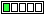All about flooble | fun stuff | Get a free chatterbox | Free JavaScript | Avatarsperplexus dot infoPolynomial and exponential (Posted on 2023-09-15)Determine all pairs of positive integers (x, y) such that x4 - 6x2 + 1 = 7·2y

 No Solution Yet Submitted by Danish Ahmed Khan No RatingComments: ( Back to comment list | You must be logged in to post comments.)Another solution Comment 3 of 3 |Take the equation mod 4.  The left side is congruent to 0 when x is odd and congruent to 1 when x is even.  The right side is congruent to 0 when y>=2, congruent to 2 when y=1 and congruent to 3 when y=0.
The only way for both sides to match is when x is odd an y>=2.

Factor x^4-6x^2+1 into (x^2+2x-1)*(x^2-2x-1).  Now substitute x=2w+1 and y=z+2 into the factored version of the equation (w and z will be nonnegative integers):
((2w+1)^2+2*(2w+1)-1)*((2w+1)^2+2*(2w+1)-1) = 7*2^(yz+2)
This simplifies to:
(2w^2+4w+1)*(2w^2-1) = 7*2^z

The left side is always odd, thus we must have z=0.  Then the two terms of the left are a factorization of 7; but there is only one factorization over positive integers: 7*1.
Then we must have 2w^2+4w+1=7 and 2w^2-1=1.  w=1 is the only common root of these equations.

With z=0 and w=1 then x=3 and y=2.  The only pair of positive integers (x,y) which satisfy the problem's equation is (3,2).

 Posted by Brian Smith on 2023-09-15 23:00:26Please log in:

 Search: Search body:
Forums (0)